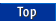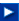Apache C++ Standard Library User's Guide

## 20.2 Creating and Using Complex Numbers

In the following sections we describe the operations used to create and manipulate complex numbers.

### 20.2.1 Declaring Complex Numbers

The template argument is used to define the types associated with the real and imaginary fields. This argument must be one of the floating point number datatypes available in the C++ language, either float, double, or long double.

There are several constructors associated with the class. A constructor with no arguments initializes both the real and imaginary fields to zero. A constructor with a single argument initializes the real field to the given value, and the imaginary value to zero. A constructor with two arguments initializes both real and imaginary fields. Finally, a copy constructor can be used to initialize a complex number with values derived from another complex number.

```std::complex<double> com_one;              // value 0 + 0i
std::complex<double> com_two(3.14);        // value 3.14 + 0i
std::complex<double> com_three(1.5, 3.14)  // value 1.5 + 3.14i
std::complex<double> com_four(com_two);    // value is also 3.14 + 0i
```

A complex number can be assigned the value of another complex number. Since the one-argument constructor is also used for a conversion operator, a complex number can also be assigned the value of a real number. The real field is changed to the right-hand side, while the imaginary field is set to zero:

```com_one = com_three;                   // becomes 1.5 + 3.14i
com_three = 2.17;                      // becomes 2.17 + 0i
```

The function polar() can be used to construct a complex number with the given magnitude and phase angle:

```com_four = std::polar(5.6, 1.8);
```

The conjugate of a complex number is formed using the function conj(). If a complex number represents x + iy, then the conjugate is the value x-iy.

```std::complex<double> com_five = std::conj(com_four);
```

### 20.2.2 Accessing Complex Number Values

The member functions real() and imag() return the real and imaginary fields of a complex number, respectively. These functions can also be invoked as ordinary functions with complex number arguments.

```// the following should be the same
std::cout << com_one.real() << "+" << com_one.imag()
<< "i" << std::endl;
std::cout << std::real(com_one) << "+" << std::imag(com_one)
<< "i" << std::endl;
```

NOTE -- With the exception of the member functions real() and imag(), most operations on complex numbers are performed using ordinary functions, not member functions.

### 20.2.3 Arithmetic Operations

The arithmetic operators +, -, *, and / can be used to perform addition, subtraction, multiplication, and division of complex numbers. All four work either with two complex numbers, or with a complex number and a real value. Assignment operators are also defined for all four.

```std::cout << com_one + com_two << std::endl;
std::cout << com_one - 3.14 << std::endl;
std::cout << 2.75 * com_two << std::endl;
com_one += com_three / 2.0;
```

The unary operators + and - can also be applied to complex numbers.

### 20.2.4 Comparing Complex Values

Two complex numbers can be compared for equality or inequality, using operator==() and operator!=(). Two values are equal if their corresponding fields are equal. Complex numbers do not have a natural ordering, and thus cannot be compared using any other relational operator.

### 20.2.5 Stream Input and Output

Complex numbers can be written to an output stream, or read from an input stream, using the normal stream I/O conventions. A value is written in parentheses, either as (u) or (u,v), depending upon whether or not the imaginary value is zero. A value is read as a set of parentheses surrounding two numeric values.

### 20.2.6 Norm and Absolute Value

The function std::norm() returns the norm of the complex number. This is the sum of the squares of the real and imaginary parts. The function std::abs() returns the absolute value, which is the square root of the norm. Note that both are ordinary functions that take the complex value as an argument, not member functions.

```std::cout << std::norm(com_two) << std::endl;
std::cout << std::abs(com_two)  << std::endl;
```

The directed phase angle of a complex number is yielded by the function std::arg():

```std::cout << com_four << " in polar coordinates is "
<< std::arg(com_four) << " and " << std::norm(com_four)
<< std::endl;
```

### 20.2.7 Trigonometric Functions

The trigonometric functions defined for floating point values have all been extended to complex number arguments. These functions are std::sin(), std::cos(), std::tan(), std::sinh(), std::cosh(), and std::tanh(). Each takes a single complex number as argument and returns a complex number as result.

### 20.2.8 Transcendental Functions

The transcendental functions std::exp(), std::log(), std::log10(), and std::sqrt() have been extended to complex arguments. Each takes a single complex number as argument, and returns a complex number as result.

The C++ Standard Library defines several variations of the exponential function std::pow(). Versions exist to raise a complex number to an integer power, to raise a complex number to a complex power or to a real power, or to raise a real value to a complex power.